Courses

Practice Test: HCF & LCM

10 Questions MCQ Test UPSC Prelims Paper 2 CSAT - Quant, Verbal & Decision Making | Practice Test: HCF & LCM

Description
Attempt Practice Test: HCF & LCM | 10 questions in 20 minutes | Mock test for UPSC preparation | Free important questions MCQ to study UPSC Prelims Paper 2 CSAT - Quant, Verbal & Decision Making for UPSC Exam | Download free PDF with solutions
QUESTION: 1

The product of two numbers is 4107. If the H.C.F. of these numbers is 37, then the greater number is:

Solution:

Let the numbers be 37a and 37b.

Then, 37a x 37b = 4107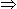ab = 3.

Now, co-primes with product 3 are (1, 3).

So, the required numbers are (37 x 1, 37 x 3) i.e., (37, 111).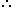Greater number = 111.

QUESTION: 2

Q. Find the greatest number that will divide 43, 91 and 183 so as to leave the same remainder in each case.

Solution:

Given numbers are 43, 91, and 183.
Subtract smallest number from both the highest numbers.
We have three cases:
183 > 43; 183 > 91 and 91 > 43
Therrefore,
183 – 43 = 140
183 – 91 = 92
91 – 43 = 48
Now, we have three new numbers: 140, 48 and 92.

HCF (140, 48 and 92) = 4
The highest number that divides 183, 91, and 43 and leaves the same remainder is 4.

QUESTION: 3

The H.C.F. of two numbers is 23 and the other two factors of their L.C.M. are 13 and 14. The larger of the two numbers is:

Solution: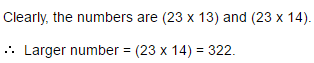QUESTION: 4

Let N be the greatest number that will divide 1305, 4665 and 6905, leaving the same remainder in each case. Then sum of the digits in N is:

Solution:

N = greatest number that will divide 105, 4665 and 6905 leaving the same remainder in each case

⇒ N = H.C.F. of (4665 - 1305), (6905 - 4665) and (6905 - 1305)
⇒ N = H.C.F. of 3360, 2240 and 5600 = 1120
Sum of digits in N = (1 + 1 + 2 + 0) = 4

QUESTION: 5

The greatest number of four digits which is divisible by 15, 25, 40 and 75 is:

Solution:

Greatest number of 4-digits is 9999.

L.C.M. of 15, 25, 40 and 75 is 600.

On dividing 9999 by 600, the remainder is 399.Required number (9999 - 399) = 9600.

QUESTION: 6

Six bells commence tolling together and toll at intervals of 2, 4, 6, 8 10, and 12 seconds respectively. In 30 minutes, how many times do they toll together?

Solution: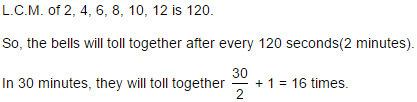QUESTION: 7

Three number are in the ratio of 3 : 4 : 5 and their L.C.M. is 2400. Their H.C.F. is:

Solution: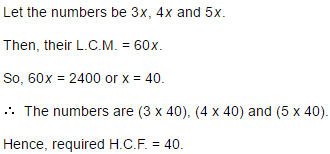QUESTION: 8

The Greatest Common Divisor of 1.08, 0.36 and 0.9 is:

Solution:

Given numbers are 1.08 , 0.36 and 0.90
G.C.D. i.e. H.C.F of 108, 36 and 90 is 18
Therefore, H.C.F of given numbers = 0.18

QUESTION: 9

The product of two numbers is 2028 and their H.C.F. is 13. The number of such pairs is:

Solution:

Let the numbers 13a and 13b.

Then, 13a x 13b = 2028

⇒ ab = 12.

Two integers a and b are said to be coprime or relatively prime if they have no common positive factor other than 1 or, equivalently, if their greatest common divisor is 1
Now, the co-primes with product 12 are (1, 12) and (3, 4).

⇒ The required numbers are (13 x 1, 13 x 12) and (13 x 3, 13 x 4).

QUESTION: 10

The least multiple of 7, which leaves a remainder of 4, when divided by 6, 9, 15 and 18 is:

Solution:

L.C.M. of 6, 9, 15 and 18 is 90.
Let required number be 90k + 4, which is multiple of 7.
Least value of k for which (90k + 4) is divisible by 7 is k = 4.
∴ Required number = (90 x 4) + 4 = 364.Use Code STAYHOME200 and get INR 200 additional OFF Use Coupon Code

How to Prepare for UPSC

Read our guide to prepare for UPSC which is created by Toppers & the best Teachers

Track your progress, build streaks, highlight & save important lessons and more!

Similar ContentRelated tests